×#### Thank you for registering.

One of our academic counsellors will contact you within 1 working day.

Click to Chat

1800-1023-196

+91-120-4616500

CART 0

• 0

MY CART (5)

Use Coupon: CART20 and get 20% off on all online Study Material

ITEM
DETAILS
MRP
DISCOUNT
FINAL PRICE
Total Price: Rs.

There are no items in this cart.
Continue Shopping• Complete JEE Main/Advanced Course and Test Series
• OFFERED PRICE: Rs. 15,900
• View Details

```Chapter 33: Probability – Exercise 33.3

Probability – Exercise 33.3 – Q.1

(i) It is valid as each p(w1) lies between 0 to 1 and sum of p(w1) = 1

(ii) It is valid as each p(w1) lies between 0 to 1 and sum of p(w1) = 1

(iii) It is not valid as sum of p(w1) = 2.8 ≠1

(iv) It is not valid as p(w7) = 15/14>1

Which is impossible

(i), (ii)

Probability – Exercise 33.3 – Q.2

(i) ∵ a die is thrown

Let E be the event of getting prime number

∴ E = {2, 3, 5}

n(E) = 3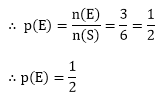(ii) E = {2, 4} ∴ n(E) = 2(iii) E = {2, 4, 6, 3}

⟹ n(E) = 4Probability – Exercise 33.3 – Q.3

Since a pair of dice have been thrown

∴ Number of elementary events in sample space is 62 = 36

(i) Let E be the event that the sum 8 appear on the faces of dice

∴ E = {(2, 6), (3, 5), (4, 9), (5, 3), (6, 2)}

∴ n(E) = 5(ii) a doublet

Let E be the event that a doublet appears on the faces of dice

∴ E = {(1, 1), (2, 2), (3, 3), (4, 4), (5, 5), (6, 6)}

⟹ n(E) = 6(iii) a doublet of prime numbers

Let E be the event that a doublet of prime number appear.

∴E = {(2, 2), (3, 3), (5, 5)}

n(E) = 3(iv) a doublet of odd numbers

Let E be the event that a doublet of odd numbers appear.

∴E = {(1, 1), (3, 3), (5, 5)}

⟹ n(E) = 3(v) a sum greater than 9

Let E be the event that a sum greater than appear

∴E = {(4, 6), (5, 5), (5, 6), (6, 4), (6, 5), (6, 6)}

∴ n(E) = 6(vi) an even number on first

Let E be the event that an even number on the first dice appear

Which means any number can be appear on second dice,∴ n(E) = 18(vii) an even number on one and a multiple of 3 on the other.

Let E be the event that an even number on one and multiple of 3 on the other appears.

∴ E = {(2,3), (2, 6), (4, 3), (4, 6), (6, 3), (6, 6), (3, 2), (3, 4) (3, 6), (6, 2), (6, 4)}

∴ n(E) = 11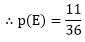be the event that either 9 or 11 as the sum of number appear on the faces of dice.= {(3, 6), (4, 5), (5, 4), (5, 6), (6, 3), (6, 5)}(ix) a sum less than 6.

Let E be the event that less than 6 as a sum offer on the faces of dice.

∴ E= {(1,1), (1, 2), (1, 3), (1, 4), (2,1), (2, 2), (2, 3), (3, 1), (3, 2), (4, 1)}

∴ n(E) = 10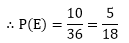(x) a sum less than 7.

Let E be the event that less than 7 as a sum appears on the faces of dice.n(E) = 15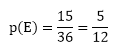(xi) a sum more than 7.

Let E be the event that a sum more than 7 appear on the faces of dice.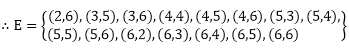⟹ n(E) = 15(xii) neither a doublet nor a total of 10.

Let E be the event that neither a doublet nor a sum of 10 appear on the faces of dice.be the event that either a doublet or a sum of 10 appear on the faces of dice.{(1, 1), (2, 2), (3, 3), (4, 6), (5, 5), (6, 4), (6, 6)}(xiii) odd number on the first and 6 on the second.

Let E be the event that an odd number on the first and 6 on the second appear on the faces of dice.

∴ E = {(1, 6), (3, 6), (5, 6)}

n(E) = 3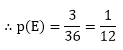(xiv) a number greater than 4 on each die.

Let E be the event that a number greater than 4 appear on each dice

∴ E = {(5, 5), (5, 6), (6, 5), (6, 6)}

⟹ n(E) = 4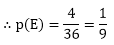(xv) a total of 9 or 11.

Let E be the event that a total of 9 or 11 appear on faces of dice.

∴ E = {(3, 6), (4, 5), (5, 4), (5, 6), (6, 3), (6, 5)}

⟹ n(E) = 6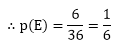(xvi) a total greater than 8.

Let E be the event that sum greater than 8 appear.

∴ E = {(3, 6), (4, 5), (4, 6), (5, 4), (5, 5), (5, 6), (6, 3), (6, 4), (6, 5), (6, 6)}

∴ n(E) = 10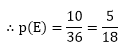Probability – Exercise 33.3 – Q.4

∵ Three dice are thrown

∴ n(S) = 63 = 216

Let E be the event of getting total of if 17 or 18

∴ E = {(6, 6, 5), (6, 5, 6), (5, 6, 6), (6, 6, 6)}

⟹ n(E) = 4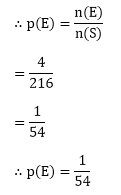Probability – Exercise 33.3 – Q.5

Three coins are tossed

∴ n(S) = 23 = 8

(i) E be the event of getting exactly two heads

∴ E = {HHT, HTH, THH}

∴ n (E) = 3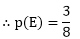∴ E = {HHH, HHT, THH, HTH}

n(E) = 4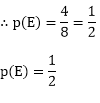(iii) at least one head and one tail

∴ E = {HTT, THT, TTH, HHT, HTH, THH}

∴ n(E) = 6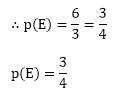```### Course Features

• 728 Video Lectures
• Revision Notes
• Previous Year Papers
• Mind Map
• Study Planner
• NCERT Solutions
• Discussion Forum
• Test paper with Video Solution